# Data Quality for Machine Learning

An illustrative look at the importance of data quality in machine learning.Goku Mohandas
· ·
Repository · Notebook

📬  Receive new lessons straight to your inbox (once a month) and join 30K+ developers in learning how to responsibly deliver value with ML.

## Overview

In a nutshell, a machine learning model consumes input data and produces predictions. The quality of the predictions directly corresponds to the quality of data you train the model with; garbage in, garbage out. Check out this article on where it makes sense to use AI and how to properly apply it.

We're going to go through all the concepts with concrete code examples and some synthesized data to train our models on. The task is to determine whether a tumor will be benign (harmless) or malignant (harmful) based on leukocyte (white blood cells) count and blood pressure. This is a synthetic dataset that we created and has no clinical relevance.

## Set up

We'll set our seeds for reproducibility.

 1 2 import numpy as np import random 
 1 SEED = 1234 
 1 2 3 # Set seed for reproducibility np.random.seed(SEED) random.seed(SEED) 

## Full dataset

We'll first train a model with the entire dataset. Later we'll remove a subset of the dataset and see the effect it has on our model.

 1 2 3 import matplotlib.pyplot as plt import pandas as pd from pandas.plotting import scatter_matrix 
 1 2 3 4 5 # Load data url = "https://raw.githubusercontent.com/GokuMohandas/MadeWithML/main/datasets/tumors.csv" df = pd.read_csv(url, header=0) # load df = df.sample(frac=1).reset_index(drop=True) # shuffle df.head() 

leukocyte_count blood_pressure tumor_class
0 15.335860 14.637535 benign
1 9.857535 14.518942 malignant
2 17.632579 15.869585 benign
3 18.369174 14.774547 benign
4 14.509367 15.892224 malignant
 1 2 3 4 5 # Define X and y X = df[["leukocyte_count", "blood_pressure"]].values y = df["tumor_class"].values print ("X: ", np.shape(X)) print ("y: ", np.shape(y)) 
X:  (1000, 2)
y:  (1000,)

 1 2 3 4 5 6 7 # Plot data colors = {"benign": "red", "malignant": "blue"} plt.scatter(X[:, 0], X[:, 1], c=[colors[_y] for _y in y], s=25, edgecolors="k") plt.xlabel("leukocyte count") plt.ylabel("blood pressure") plt.legend(["malignant", "benign"], loc="upper right") plt.show()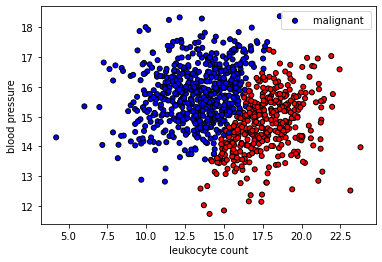We want to choose features that have strong predictive signal for our task. If you want to improve performance, you need to continuously do feature engineering by collecting and adding new signals. So you may run into a new feature that has high correlation (orthogonal signal) with your existing features but it may still possess some unique signal to boost your predictive performance.

 1 2 3 # Correlation matrix scatter_matrix(df, figsize=(5, 5)); df.corr() 

leukocyte_count blood_pressure
leukocyte_count 1.000000 -0.162875
blood_pressure -0.162875 1.000000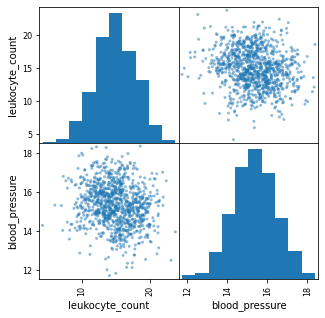### Split data

 1 2 import collections from sklearn.model_selection import train_test_split 
 1 2 3 TRAIN_SIZE = 0.70 VAL_SIZE = 0.15 TEST_SIZE = 0.15 
 1 2 3 4 5 def train_val_test_split(X, y, train_size): """Split dataset into data splits.""" X_train, X_, y_train, y_ = train_test_split(X, y, train_size=TRAIN_SIZE, stratify=y) X_val, X_test, y_val, y_test = train_test_split(X_, y_, train_size=0.5, stratify=y_) return X_train, X_val, X_test, y_train, y_val, y_test 
 1 2 3 4 5 6 7 # Create data splits X_train, X_val, X_test, y_train, y_val, y_test = train_val_test_split( X=X, y=y, train_size=TRAIN_SIZE) print (f"X_train: {X_train.shape}, y_train: {y_train.shape}") print (f"X_val: {X_val.shape}, y_val: {y_val.shape}") print (f"X_test: {X_test.shape}, y_test: {y_test.shape}") print (f"Sample point: {X_train} → {y_train}") 

X_train: (700, 2), y_train: (700,)
X_val: (150, 2), y_val: (150,)
X_test: (150, 2), y_test: (150,)
Sample point: [11.5066204  15.98030799] → malignant


### Label encoding

 1 from sklearn.preprocessing import LabelEncoder 
 1 2 # Output vectorizer label_encoder = LabelEncoder() 
 1 2 3 4 # Fit on train data label_encoder = label_encoder.fit(y_train) classes = list(label_encoder.classes_) print (f"classes: {classes}") 

classes: ["benign", "malignant"]

 1 2 3 4 5 6 # Convert labels to tokens print (f"y_train: {y_train}") y_train = label_encoder.transform(y_train) y_val = label_encoder.transform(y_val) y_test = label_encoder.transform(y_test) print (f"y_train: {y_train}") 
y_train: malignant
y_train: 1

 1 2 3 4 # Class weights counts = np.bincount(y_train) class_weights = {i: 1.0/count for i, count in enumerate(counts)} print (f"counts: {counts}\nweights: {class_weights}") 
counts: [272 428]
weights: {0: 0.003676470588235294, 1: 0.002336448598130841}


### Standardize data

 1 from sklearn.preprocessing import StandardScaler 
 1 2 # Standardize the data (mean=0, std=1) using training data X_scaler = StandardScaler().fit(X_train) 
 1 2 3 4 # Apply scaler on training and test data (don't standardize outputs for classification) X_train = X_scaler.transform(X_train) X_val = X_scaler.transform(X_val) X_test = X_scaler.transform(X_test) 
 1 2 3 # Check (means should be ~0 and std should be ~1) print (f"X_test: mean: {np.mean(X_test[:, 0], axis=0):.1f}, std: {np.std(X_test[:, 0], axis=0):.1f}") print (f"X_test: mean: {np.mean(X_test[:, 1], axis=0):.1f}, std: {np.std(X_test[:, 1], axis=0):.1f}") 

X_test: mean: 0.0, std: 1.0
X_test: mean: 0.0, std: 1.0


### Model

 1 2 3 import torch from torch import nn import torch.nn.functional as F 
 1 2 # Set seed for reproducibility torch.manual_seed(SEED) 
 1 2 3 INPUT_DIM = 2 # X is 2-dimensional HIDDEN_DIM = 100 NUM_CLASSES = 2 
  1 2 3 4 5 6 7 8 9 10 11 12 class MLP(nn.Module): def __init__(self, input_dim, hidden_dim, num_classes): super(MLP, self).__init__() self.fc1 = nn.Linear(input_dim, hidden_dim) self.fc2 = nn.Linear(hidden_dim, num_classes) def forward(self, x_in, apply_softmax=False): z = F.relu(self.fc1(x_in)) # ReLU activaton function added! y_pred = self.fc2(z) if apply_softmax: y_pred = F.softmax(y_pred, dim=1) return y_pred 
 1 2 3 # Initialize model model = MLP(input_dim=INPUT_DIM, hidden_dim=HIDDEN_DIM, num_classes=NUM_CLASSES) print (model.named_parameters) 

<bound method Module.named_parameters of MLP(
(fc1): Linear(in_features=2, out_features=100, bias=True)
(fc2): Linear(in_features=100, out_features=2, bias=True)
)>


### Training

 1 from torch.optim import Adam 
 1 2 3 LEARNING_RATE = 1e-3 NUM_EPOCHS = 5 BATCH_SIZE = 32 
 1 2 3 # Define Loss class_weights_tensor = torch.Tensor(list(class_weights.values())) loss_fn = nn.CrossEntropyLoss(weight=class_weights_tensor) 
 1 2 3 4 5 # Accuracy def accuracy_fn(y_pred, y_true): n_correct = torch.eq(y_pred, y_true).sum().item() accuracy = (n_correct / len(y_pred)) * 100 return accuracy 
 1 2 # Optimizer optimizer = Adam(model.parameters(), lr=LEARNING_RATE) 
 1 2 3 4 5 6 7 # Convert data to tensors X_train = torch.Tensor(X_train) y_train = torch.LongTensor(y_train) X_val = torch.Tensor(X_val) y_val = torch.LongTensor(y_val) X_test = torch.Tensor(X_test) y_test = torch.LongTensor(y_test) 
  1 2 3 4 5 6 7 8 9 10 11 12 13 14 15 16 17 18 19 20 21 # Training for epoch in range(NUM_EPOCHS*10): # Forward pass y_pred = model(X_train) # Loss loss = loss_fn(y_pred, y_train) # Zero all gradients optimizer.zero_grad() # Backward pass loss.backward() # Update weights optimizer.step() if epoch%10==0: predictions = y_pred.max(dim=1) # class accuracy = accuracy_fn(y_pred=predictions, y_true=y_train) print (f"Epoch: {epoch} | loss: {loss:.2f}, accuracy: {accuracy:.1f}") 

Epoch: 0 | loss: 0.70, accuracy: 49.6
Epoch: 10 | loss: 0.54, accuracy: 93.7
Epoch: 20 | loss: 0.43, accuracy: 97.1
Epoch: 30 | loss: 0.35, accuracy: 97.0
Epoch: 40 | loss: 0.30, accuracy: 97.4


### Evaluation

 1 2 3 import json import matplotlib.pyplot as plt from sklearn.metrics import precision_recall_fscore_support 
  1 2 3 4 5 6 7 8 9 10 11 12 13 14 15 16 17 18 19 20 21 22 23 def get_metrics(y_true, y_pred, classes): """Per-class performance metrics.""" # Performance performance = {"overall": {}, "class": {}} # Overall performance metrics = precision_recall_fscore_support(y_true, y_pred, average="weighted") performance["overall"]["precision"] = metrics performance["overall"]["recall"] = metrics performance["overall"]["f1"] = metrics performance["overall"]["num_samples"] = np.float64(len(y_true)) # Per-class performance metrics = precision_recall_fscore_support(y_true, y_pred, average=None) for i in range(len(classes)): performance["class"][classes[i]] = { "precision": metrics[i], "recall": metrics[i], "f1": metrics[i], "num_samples": np.float64(metrics[i]), } return performance 
 1 2 3 # Predictions y_prob = model(X_test, apply_softmax=True) y_pred = y_prob.max(dim=1) 
 1 2 3 # Performance report performance = get_metrics(y_true=y_test, y_pred=y_pred, classes=classes) print (json.dumps(performance, indent=2)) 

{
"overall": {
"precision": 0.9461538461538461,
"recall": 0.9619565217391304,
"f1": 0.9517707041477195,
"num_samples": 150.0
},
"class": {
"benign": {
"precision": 0.8923076923076924,
"recall": 1.0,
"f1": 0.9430894308943091,
"num_samples": 58.0
},
"malignant": {
"precision": 1.0,
"recall": 0.9239130434782609,
"f1": 0.96045197740113,
"num_samples": 92.0
}
}
}


### Inference

We're going to plot a point, which we know belongs to the malignant tumor class. Our well trained model here would accurately predict that it is indeed a malignant tumor!

  1 2 3 4 5 6 7 8 9 10 11 12 13 14 def plot_multiclass_decision_boundary(model, X, y): x_min, x_max = X[:, 0].min() - 0.1, X[:, 0].max() + 0.1 y_min, y_max = X[:, 1].min() - 0.1, X[:, 1].max() + 0.1 xx, yy = np.meshgrid(np.linspace(x_min, x_max, 101), np.linspace(y_min, y_max, 101)) cmap = plt.cm.Spectral X_test = torch.from_numpy(np.c_[xx.ravel(), yy.ravel()]).float() y_pred = model(X_test, apply_softmax=True) _, y_pred = y_pred.max(dim=1) y_pred = y_pred.reshape(xx.shape) plt.contourf(xx, yy, y_pred, cmap=plt.cm.Spectral, alpha=0.8) plt.scatter(X[:, 0], X[:, 1], c=y, s=40, cmap=plt.cm.RdYlBu) plt.xlim(xx.min(), xx.max()) plt.ylim(yy.min(), yy.max()) 
  1 2 3 4 5 6 7 8 9 10 11 12 13 14 15 16 17 18 19 20 # Visualize the decision boundary plt.figure(figsize=(8,5)) plt.title("Test") plot_multiclass_decision_boundary(model=model, X=X_test, y=y_test) # Sample point near the decision boundary mean_leukocyte_count, mean_blood_pressure = X_scaler.transform( [[np.mean(df.leukocyte_count), np.mean(df.blood_pressure)]]) plt.scatter(mean_leukocyte_count+0.05, mean_blood_pressure-0.05, s=200, c="b", edgecolor="w", linewidth=2) # Annotate plt.annotate("true: malignant,\npred: malignant", color="white", xy=(mean_leukocyte_count, mean_blood_pressure), xytext=(0.4, 0.65), textcoords="figure fraction", fontsize=16, arrowprops=dict(facecolor="white", shrink=0.1)) plt.show()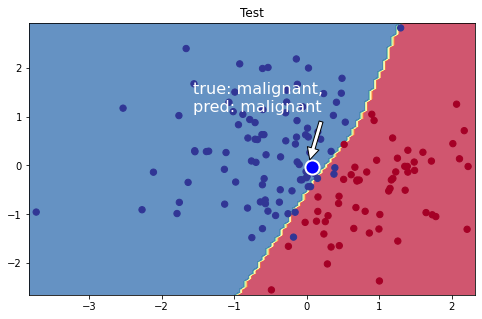Great! We received great performances on both our train and test data splits. We're going to use this dataset to show the importance of data quality.

## Reduced dataset

Let's remove some training data near the decision boundary and see how robust the model is now.

 1 2 3 4 5 # Raw reduced data url = "https://raw.githubusercontent.com/GokuMohandas/MadeWithML/main/datasets/tumors_reduced.csv" df_reduced = pd.read_csv(url, header=0) # load df_reduced = df_reduced.sample(frac=1).reset_index(drop=True) # shuffle df_reduced.head() 
leukocyte_count blood_pressure tumor_class
0 16.795186 14.434741 benign
1 13.472969 15.250393 malignant
2 9.840450 16.434717 malignant
3 16.390730 14.419258 benign
4 13.367974 15.741790 malignant
 1 2 3 4 5 # Define X and y X = df_reduced[["leukocyte_count", "blood_pressure"]].values y = df_reduced["tumor_class"].values print ("X: ", np.shape(X)) print ("y: ", np.shape(y)) 
X:  (720, 2)
y:  (720,)

 1 2 3 4 5 6 7 # Plot data colors = {"benign": "red", "malignant": "blue"} plt.scatter(X[:, 0], X[:, 1], c=[colors[_y] for _y in y], s=25, edgecolors="k") plt.xlabel("leukocyte count") plt.ylabel("blood pressure") plt.legend(["malignant", "benign"], loc="upper right") plt.show()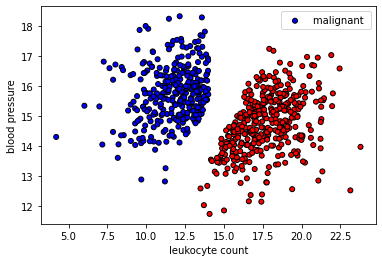### Split data

 1 2 3 4 5 6 7 # Create data splits X_train, X_val, X_test, y_train, y_val, y_test = train_val_test_split( X=X, y=y, train_size=TRAIN_SIZE) print (f"X_train: {X_train.shape}, y_train: {y_train.shape}") print (f"X_val: {X_val.shape}, y_val: {y_val.shape}") print (f"X_test: {X_test.shape}, y_test: {y_test.shape}") print (f"Sample point: {X_train} → {y_train}") 
X_train: (503, 2), y_train: (503,)
X_val: (108, 2), y_val: (108,)
X_test: (109, 2), y_test: (109,)
Sample point: [19.66235758 15.65939541] → benign


### Label encoding

 1 2 3 4 5 6 7 # Encode class labels label_encoder = LabelEncoder() label_encoder = label_encoder.fit(y_train) num_classes = len(label_encoder.classes_) y_train = label_encoder.transform(y_train) y_val = label_encoder.transform(y_val) y_test = label_encoder.transform(y_test) 
 1 2 3 4 # Class weights counts = np.bincount(y_train) class_weights = {i: 1.0/count for i, count in enumerate(counts)} print (f"counts: {counts}\nweights: {class_weights}") 

counts: [272 231]
weights: {0: 0.003676470588235294, 1: 0.004329004329004329}


### Standardize data

 1 2 3 4 5 # Standardize inputs using training data X_scaler = StandardScaler().fit(X_train) X_train = X_scaler.transform(X_train) X_val = X_scaler.transform(X_val) X_test = X_scaler.transform(X_test) 

### Model

 1 2 # Initialize model model = MLP(input_dim=INPUT_DIM, hidden_dim=HIDDEN_DIM, num_classes=NUM_CLASSES) 

### Training

 1 2 3 # Define Loss class_weights_tensor = torch.Tensor(list(class_weights.values())) loss_fn = nn.CrossEntropyLoss(weight=class_weights_tensor) 
 1 2 # Optimizer optimizer = Adam(model.parameters(), lr=LEARNING_RATE) 
 1 2 3 4 5 6 7 # Convert data to tensors X_train = torch.Tensor(X_train) y_train = torch.LongTensor(y_train) X_val = torch.Tensor(X_val) y_val = torch.LongTensor(y_val) X_test = torch.Tensor(X_test) y_test = torch.LongTensor(y_test) 
  1 2 3 4 5 6 7 8 9 10 11 12 13 14 15 16 17 18 19 20 21 # Training for epoch in range(NUM_EPOCHS*10): # Forward pass y_pred = model(X_train) # Loss loss = loss_fn(y_pred, y_train) # Zero all gradients optimizer.zero_grad() # Backward pass loss.backward() # Update weights optimizer.step() if epoch%10==0: predictions = y_pred.max(dim=1) # class accuracy = accuracy_fn(y_pred=predictions, y_true=y_train) print (f"Epoch: {epoch} | loss: {loss:.2f}, accuracy: {accuracy:.1f}") 

Epoch: 0 | loss: 0.68, accuracy: 69.8
Epoch: 10 | loss: 0.53, accuracy: 99.6
Epoch: 20 | loss: 0.42, accuracy: 99.6
Epoch: 30 | loss: 0.33, accuracy: 99.6
Epoch: 40 | loss: 0.27, accuracy: 99.8


### Evaluation

 1 2 3 # Predictions y_prob = model(X_test, apply_softmax=True) y_pred = y_prob.max(dim=1) 
 1 2 3 # Performance report performance = get_metrics(y_true=y_test, y_pred=y_pred, classes=classes) print (json.dumps(performance, indent=2)) 

{
"overall": {
"precision": 1.0,
"recall": 1.0,
"f1": 1.0,
"num_samples": 109.0
},
"class": {
"benign": {
"precision": 1.0,
"recall": 1.0,
"f1": 1.0,
"num_samples": 59.0
},
"malignant": {
"precision": 1.0,
"recall": 1.0,
"f1": 1.0,
"num_samples": 50.0
}
}
}


### Inference

Now let's see how the same inference point from earlier performs now on the model trained on the reduced dataset.

  1 2 3 4 5 6 7 8 9 10 11 12 13 14 15 16 17 18 # Visualize the decision boundary plt.figure(figsize=(8,5)) plt.title("Test") plot_multiclass_decision_boundary(model=model, X=X_test, y=y_test) # Sample point near the decision boundary (same point as before) plt.scatter(mean_leukocyte_count+0.05, mean_blood_pressure-0.05, s=200, c="b", edgecolor="w", linewidth=2) # Annotate plt.annotate("true: malignant,\npred: benign", color="white", xy=(mean_leukocyte_count, mean_blood_pressure), xytext=(0.45, 0.60), textcoords="figure fraction", fontsize=16, arrowprops=dict(facecolor="white", shrink=0.1)) plt.show()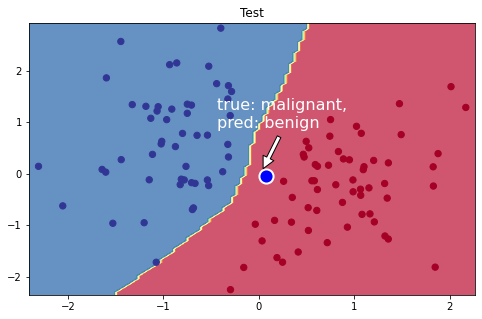This is a very fragile but highly realistic scenario. Based on our reduced synthetic dataset, we have achieved a model that generalized really well on the test data. But when we ask for the prediction for the same point tested earlier (which we known is malignant), the prediction is now a benign tumor. We would have completely missed the tumor. To mitigate this, we can:

1. Get more data around the space we are concerned about
2. Consume predictions with caution when they are close to the decision boundary

## Takeaway

Models are not crystal balls. So it's important that before any machine learning, we really look at our data and ask ourselves if it is truly representative for the task we want to solve. The model itself may fit really well and generalize well on your data but if the data is of poor quality to begin with, the model cannot be trusted.

Once you are confident that your data is of good quality, you can finally start thinking about modeling. The type of model you choose depends on many factors, including the task, type of data, complexity required, etc.

 1 2 3 4 5 6 @article{madewithml, author = {Goku Mohandas}, title = { Data quality - Made With ML }, howpublished = {\url{https://madewithml.com/}}, year = {2021} }